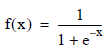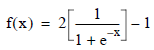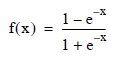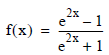# Sigmoid - Teradata Warehouse Miner

Product
Release Number
5.4.6
Published
November 2018
Language
English (United States)
Last Update
2018-12-07
dita:mapPath
gxn1538171534877.ditamap
dita:ditavalPath
ft:empty
dita:id
B035-2301
Product Category
Software

A Sigmoid transformation provides rescaling of continuous numeric data in a more sophisticated way than the Rescaling transformation function. In a Sigmoid transformation, a numeric column is transformed using a type of sigmoid or s-shaped function. One of these, called a logit function, produces a continuously increasing value between 0 and 1. Another called the modified logit function, is twice the logit minus 1 and produces a value between -1 and 1. A third, called the hyperbolic tangent function, also produces a value between -1 and 1.

Note that the logit function is the same as the function previously called the sigmoid function, and the hyperbolic tangent function is the same as the math function of the same name. These non-linear transformations are generally more useful in data mining than a linear Rescaling transformation.

The logit value is calculated as:The modified logit value is calculated as:which is equivalent to:The hyperbolic tangent value is calculated as:For absolute values of x greater than or equal to 36, the value of the sigmoid function is effectively 1 for positive arguments or 0 for negative arguments, within about 15 digits of significance.